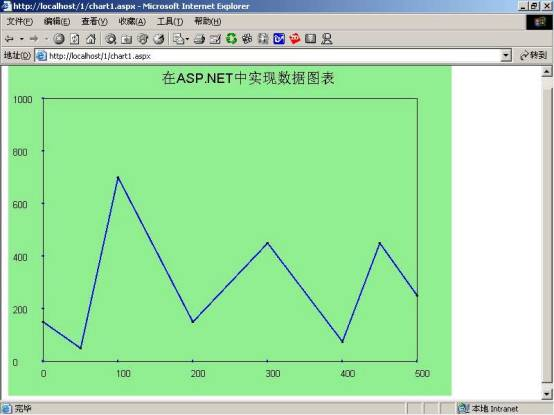## ASP.NET实现数据图表

（1）.微软视窗2000 服务器版

（2）..Net Framework SDK Beta 2以上版本

（1）.首先我们来看看如何在ASP.NET页面创建一个动态图片，并显示出来。

 `//创建一个"Bitmap"对象Bitmap image = new Bitmap ( 400 , 400 ) ;`

 `//以"Jpeg"格式保存此图片对象，在客户端显示出来image . Save ( Response . OutputStream , ImageFormat . Jpeg ); `

 `//以"Jpeg"格式保存此图片对象，在客户端显示出来image . Save ( Response . OutputStream , ImageFormat . Gif ) ;`

 `＜%@ Page Language = "C#" ContentType = "image/jpeg" %＞ ＜%@ Import Namespace = "System" %＞＜%@ Import Namespace = "System.Drawing" %＞＜%@ Import Namespace = "System.Drawing.Drawing2D" %＞＜%@ Import Namespace = "System.Drawing.Imaging" %＞＜html ＞ ＜head ＞ ＜script language = "C#" runat = "server" ＞ void Page_Load ( object sender , EventArgs e ) { //创建一个"Bitmap"对象Bitmap image = new Bitmap ( 400 , 400 ) ;//以"Jpeg"格式保存此图片对象，在客户端显示出来image . Save ( Response . OutputStream , ImageFormat . Jpeg ) ; } ＜/script ＞ ＜/head ＞ ＜body ＞ ＜/body ＞ ＜/html ＞`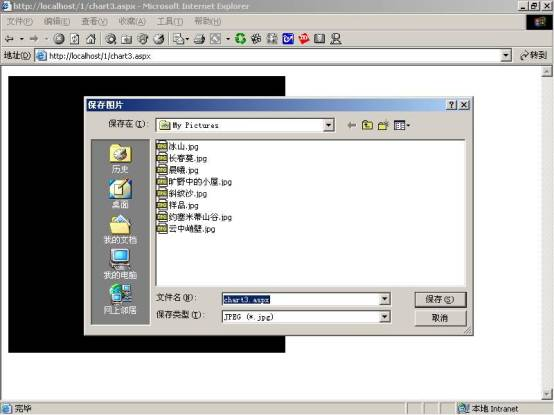图01：利用ASP.NET动态创建图片

（2）.如何给产生得图片上色：

 `＜%@ Page Language = "C#" ContentType = "image/jpeg" %＞ ＜%@ Import Namespace = "System" %＞＜%@ Import Namespace = "System.Drawing" %＞＜%@ Import Namespace = "System.Drawing.Drawing2D" %＞＜%@ Import Namespace = "System.Drawing.Imaging" %＞＜html ＞ ＜head ＞ ＜script language = "C#" runat = "server" ＞ void Page_Load ( object sender , EventArgs e ) { //创建一个"Bitmap"对象Bitmap image = new Bitmap ( 400 , 400 ) ;Graphics g = Graphics . FromImage ( image ) ; g . FillRectangle ( new SolidBrush ( Color . LightGreen ) , 0 , 0 , 400 , 400 ) ;//以"Jpeg"格式保存此图片对象，在客户端显示出来image . Save ( Response . OutputStream , ImageFormat . Jpeg ) ; } ＜/script ＞ ＜/head ＞ ＜body ＞ ＜/body ＞ ＜/html ＞`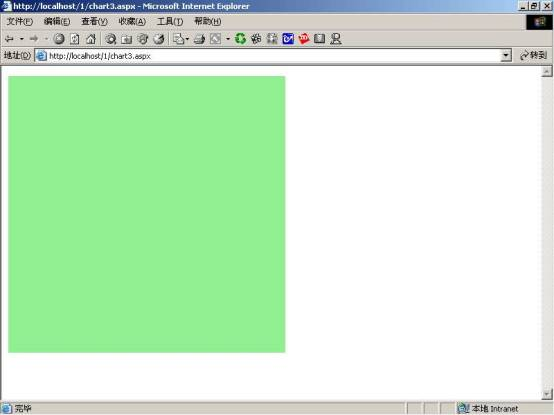图02：给产生的图片上色

 `＜%@ Page Language = "C#" ContentType = "image/jpeg" %＞ ＜%@ Import Namespace = "System" %＞＜%@ Import Namespace = "System.Drawing" %＞＜%@ Import Namespace = "System.Drawing.Drawing2D" %＞＜%@ Import Namespace = "System.Drawing.Imaging" %＞＜html ＞ ＜head ＞ ＜script language = "C#" runat = "server" ＞ void Page_Load ( object sender , EventArgs e ) { //创建一个"Bitmap"对象Bitmap image = new Bitmap ( 400 , 400 ) ;Graphics g = Graphics . FromImage ( image ) ; g . FillRectangle ( new SolidBrush ( Color . LightGreen ) , 0 , 0 , 400 , 400 ) ;//以"Jpeg"格式保存此图片对象，在客户端显示出来image . Save ( Response . OutputStream , ImageFormat . Jpeg ) ; } ＜/script ＞ ＜/head ＞ ＜body ＞ ＜/body ＞ ＜/html ＞`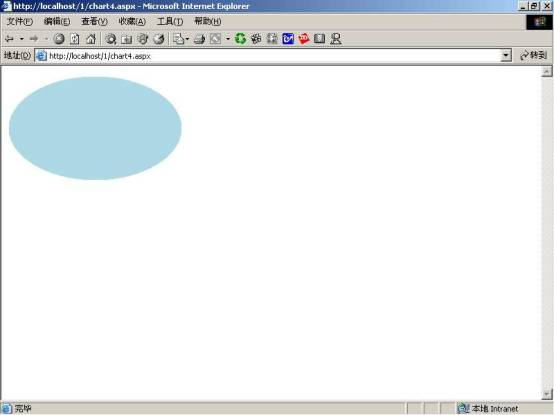图03：定制图片的形状

（3）.如何在图片上实现画线和写字：

 `public void DrawString ( string s ,Font font ,Brush brush ,float x ,float y) ;`

"s"是要输出的字符串，"font"是字符串的字体，"brush"是定义刷子，后面二个参数是产生字符串的位置坐标。在程序中产生字符串的具体语句如下：

 `Font axesFont = new Font ( "arial" , 10 ) ;Brush blackBrush = new SolidBrush ( Color . Red ) ;g . DrawString ( "在图片上面写入文字，呵呵" , axesFont , blackBrush , 90 , 20 ) ; `

 `public void DrawLines (Pen pen ,Point [ ] points) ;`

 `Pen redPen = new Pen ( Color . Red , 1 ) ;Pen blackPen = new Pen ( Color . Blue , 2 ) ;//以下语句是在此图片对象上画出各种线条，可以定义线条的粗细、起点、终点等g . DrawLine ( blackPen , 0 , 2 , 210 , 250 ) ;g . DrawLine ( blackPen , 210 , 250 , 310 , 50 ) ;g . DrawLine ( redPen , 310 , 50 , 210 , 350 ) ;`

 `＜%@ Page Language = "C#" ContentType = "image/jpeg" %＞ ＜%@ Import Namespace = "System" %＞＜%@ Import Namespace = "System.Drawing" %＞＜%@ Import Namespace = "System.Drawing.Drawing2D" %＞＜%@ Import Namespace = "System.Drawing.Imaging" %＞＜html ＞ ＜head ＞ ＜script language = "C#" runat = "server" ＞ void Page_Load ( object sender , EventArgs e ) { Bitmap image = new Bitmap ( 400 , 400 ) ;Font axesFont = new Font ( "arial" , 10 ) ;Brush blackBrush = new SolidBrush ( Color . Red ) ;Pen redPen = new Pen ( Color . Red , 1 ) ;Pen blackPen = new Pen ( Color . Blue , 2 ) ;Graphics g = Graphics . FromImage ( image ) ; g . Clear ( Color . White ) ; g . FillRectangle ( new SolidBrush ( Color . LightGreen ) , 0 , 0 , 400 , 400 ) ;//在此图片对象中画出图片,可以定义文字大小、位置、色彩等g . DrawString ( "在图片上面写入文字，呵呵" , axesFont , blackBrush , 90 , 20 ) ;//以下语句是在此图片对象上画出各种线条，可以定义线条的粗细、起点、终点等g . DrawLine ( blackPen , 0 , 2 , 210 , 250 ) ;g . DrawLine ( blackPen , 210 , 250 , 310 , 50 ) ;g . DrawLine ( redPen , 310 , 50 , 210 , 350 ) ;//以"Jpeg"格式保存此图片对象，在客户端显示出来// image . Save ( Response . OutputStream , ImageFormat . Jpeg ) ; image . Save ( Response . OutputStream , ImageFormat . Jpeg ) ; } ＜/script ＞ ＜/head ＞ ＜body ＞ ＜/body ＞ ＜/html ＞`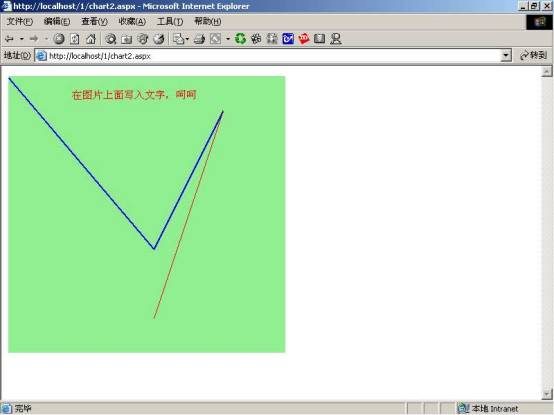图04：在图片上完成画线、写字和上色

 `＜%@ Import Namespace = "System" %＞＜%@ Import Namespace = "System.Drawing" %＞＜%@ Import Namespace = "System.Drawing.Drawing2D" %＞＜%@ Import Namespace = "System.Drawing.Imaging" %＞ ＜script language = "C#" runat = "server" ＞class LineChart{public Bitmap b ;public string Title = "在ASP.NET中实现数据图表" ;public ArrayList chartValues = new ArrayList ( ) ;public float Xorigin = 0 , Yorigin = 0 ;public float ScaleX , ScaleY ;public float Xdivs = 2 , Ydivs = 2 ;private int Width , Height ;private Graphics g ;private Page p ;struct datapoint {public float x ;public float y ;public bool valid ;}//初始化public LineChart ( int myWidth , int myHeight , Page myPage ) {Width = myWidth ; Height = myHeight ;ScaleX = myWidth ; ScaleY = myHeight ;b = new Bitmap ( myWidth , myHeight ) ;g = Graphics . FromImage ( b ) ;p = myPage ;}public void AddValue ( int x , int y ) {datapoint myPoint ;myPoint . x = x ;myPoint . y = y ;myPoint . valid = true ;chartValues . Add ( myPoint ) ;}public void Draw ( ) {int i ;float x , y , x0 , y0 ;string myLabel ;Pen blackPen = new Pen ( Color . Blue , 2 ) ;Brush blackBrush = new SolidBrush ( Color . Black ) ;Font axesFont = new Font ( "arial" , 10 ) ;//首先要创建图片的大小p . Response . ContentType = "image/jpeg" ;g . FillRectangle ( new SolidBrush ( Color . LightGreen ) , 0 , 0 , Width , Height ) ;int ChartInset = 50 ;int ChartWidth = Width - ( 2 * ChartInset ) ;int ChartHeight = Height - ( 2 * ChartInset ) ;g . DrawRectangle ( new Pen ( Color . Black , 1 ) , ChartInset , ChartInset , ChartWidth , ChartHeight ) ;//写出图片上面的图片内容文字g . DrawString ( Title , new Font ( "arial" , 14 ) , blackBrush , Width / 3 , 10 ) ;//沿X坐标写入X标签for ( i = 0 ; i ＜= Xdivs ; i++ ) {x = ChartInset + ( i * ChartWidth ) / Xdivs ;y = ChartHeight + ChartInset ;myLabel = ( Xorigin + ( ScaleX * i / Xdivs ) ) . ToString ( ) ;g . DrawString ( myLabel , axesFont , blackBrush , x - 4 , y + 10 ) ;g . DrawLine ( blackPen , x , y + 2 , x , y - 2 ) ;}//沿Y坐标写入Y标签for ( i = 0 ; i ＜= Ydivs ; i++ ){x = ChartInset ;y = ChartHeight + ChartInset - ( i * ChartHeight / Ydivs ) ;myLabel = ( Yorigin + ( ScaleY * i / Ydivs ) ) . ToString ( ) ;g . DrawString ( myLabel , axesFont , blackBrush , 5 , y - 6 ) ;g . DrawLine ( blackPen , x + 2 , y , x - 2 , y ) ;}g . RotateTransform ( 180 ) ;g . TranslateTransform ( 0 , - Height ) ;g . TranslateTransform ( - ChartInset , ChartInset ) ;g . ScaleTransform ( - 1 , 1 ) ;//画出图表中的数据datapoint prevPoint = new datapoint ( ) ;prevPoint . valid = false ;foreach ( datapoint myPoint in chartValues ) {if ( prevPoint . valid == true ) {x0 = ChartWidth * ( prevPoint . x - Xorigin ) / ScaleX ;y0 = ChartHeight * ( prevPoint . y - Yorigin ) / ScaleY ;x = ChartWidth * ( myPoint . x - Xorigin ) / ScaleX ;y = ChartHeight * ( myPoint . y - Yorigin ) / ScaleY ;g . DrawLine ( blackPen , x0 , y0 , x , y ) ;g . FillEllipse ( blackBrush , x0 - 2 , y0 - 2 , 4 , 4 ) ;g . FillEllipse ( blackBrush , x - 2 , y - 2 , 4 , 4 ) ;}prevPoint = myPoint ;}//最后以图片形式来浏览b . Save ( p . Response . OutputStream , ImageFormat . Jpeg ) ;}~LineChart ( ) {g . Dispose ( ) ;b . Dispose ( ) ;}}void Page_Load ( Object sender , EventArgs e ) {LineChart c = new LineChart ( 640 , 480 , Page ) ;c . Title = " 在ASP.NET中实现数据图表" ;c . Xorigin = 0 ; c . ScaleX = 500 ; c . Xdivs = 5 ;c . Yorigin = 0 ; c . ScaleY = 1000 ; c . Ydivs = 5 ;c . AddValue ( 0 , 150 ) ;c . AddValue ( 50 , 50 ) ;c . AddValue ( 100 , 700 ) ;c . AddValue ( 200 , 150 ) ;c . AddValue ( 300 , 450 ) ;c . AddValue ( 400 , 75 ) ;c . AddValue ( 450 , 450 ) ;c . AddValue ( 500 , 250 ) ;c . Draw ( ) ;}＜/script ＞`